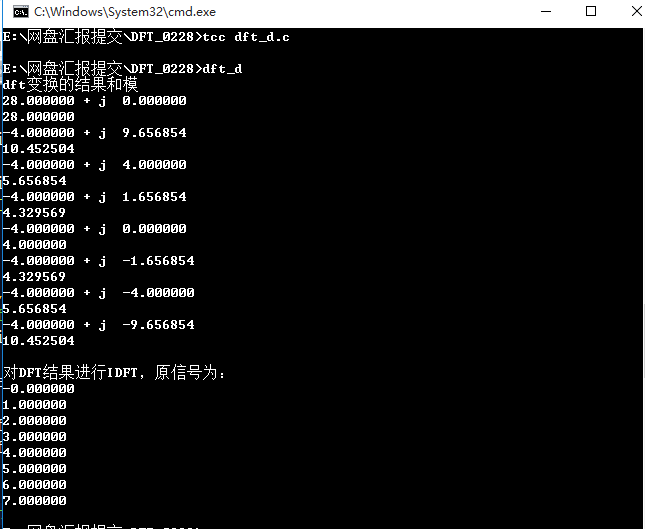• ## DFT与IDFT

万次阅读 2019-02-28 20:38:01
DFTIDFT 一.方法简介 序列x(n)(n=0,1,…N-1)的DFT定义为 X（k）=∑n=0N−1x(n)e−j2πnkN X（k）=\sum_{n=0}^{N-1}x(n)e^{-j\frac{2\pi nk}{N}} X（k）=n=0∑N−1​x(n)e−jN2πnk​ 设x(n)=a(n)+jb(n),X(k)...
DFT与IDFT
一.方法简介
序列x(n)(n=0,1,…N-1)的DFT定义为
$X（k）=\sum_{n=0}^{N-1}x(n)e^{-j\frac{2\pi nk}{N}}$
$设x(n)=a(n)+jb(n),\quad X(k)=A(k)+jB(K),\quad Q=2\pi/N$
则上式变为：
$A（k）+jB(k)=\sum_{n=0}^{N-1}[a(n)+jb(n)][\cos(Qnk)-j\sin(Qnk)]$
即
$A（k）=\sum_{n=0}^{N-1}[a(n)\cos(Qnk)+b(n)\sin(Qnk)]$
$B(k)=\sum_{n=0}^{N-1}[b(n)\cos(Qnk)-a(n)\sin(Qnk)]$
序列X（k）的IDFT定义为：
$x(n)=\frac{1}{N}\sum_{k=0}^{N-1}X(k)W_{N}^{-nk},\quad n=0,1,...N-1$
$它与DFT的区别在于将W_{N}改变为改变为W_{N}^{-1},并多了一个除以N 的运算，公式如下$
$a(n)=\frac{1}{N}\sum_{k=0}^{N-1}[A(k)\cos(Qnk)-B(k)\sin(Qnk)]$
$b(n)=\frac{1}{N}\sum_{k=0}^{N-1}[B(k)\cos(Qnk)+A(k)\sin(Qnk]$
二.编程实现
考滤到DFT和IDFT算法过程中有部分相似，可以把它们合成到一个算法。
DFT.c
/*
x-存放要变换数据的实部
y-存放要变换数据的虚部
a-存放变换结果的实部
b-存放变换结果的虚部
n-数据长度
sign-为1时执行DFT，为-1时执行IDFT
*/
#include "math.h"
void dft(x,y,a,b,n,sign)
int n, sign;
double x[],y[],a[],b[];
{
int i,k;
double c,d,q,w,s;
q = 6.28318530718/n;
for (k=0;k<n;k++)
{
w=k*q;
a[k]=b[k]=0.0;
for(i=0;i<n;i++)
{
d=i*w;
c=cos(d);
s=sin(d)*sign;
a[k]+=c*x[i] + s*y[i];
b[k]+=c*y[i] - s*x[i];
}
}
if(sign == -1)
{
c=1.0/n;
for (k=0;k<n;k++)
{
a[k]=c*a[k];
b[k]=c*b[k];
}
}
}

下面验证此算法，对X(n)=(0,1,2,3,4,5,6,7)，做DFT和IDFT算法
dft_d.c
#include "stdio.h"
#include "math.h"
#include "dft.c"
#define N 4
static double  x[N],y[N],a[N],b[N],c[N];
main(){
int k;
int i=0;
for(i=0; i<N; i++)
{
x[i]=i;
y[i]=0;

}
dft(x,y,a,b,N,1);	//DFT变换
for(i=0; i<N; i++)
{
c[i]=sqrt(a[i]*a[i]+b[i]*b[i]);	//算出模
printf("%lf + j  %lf \n",a[i],b[i]);//输出变换后结果
printf("%lf \n",c[i]); //输出模值
printf("\n");
}
dft(a,b,x,y,N,-1); //IDFT变换
for(i=0; i<N; i++)
{
printf("%lf \n",x[i]); //输出x(n)的实部
}

}

运行结果：展开全文• DFT-dftmtx 是对称 Vandermonder Matrix conj(dftmtx )=dftmtx '; IDFT-conj(dftmtx )/N fft(x)-------conj(dftmtx )*x % x column vector ifft(x)--------conj(dftmtx )/N*x % fft 和
傅里叶变换 矩阵
DFT-dftmtx 是对称 Vandermonder Matrix  conj(dftmtx )=dftmtx ';
IDFT-conj(dftmtx )/N
fft(x)-------conj(dftmtx )*x        %  x   column vector
ifft(x)--------conj(dftmtx )/N*x   %

fft 和iff 关系：
Xk=ifft(x);
Xk=conj(fft(conj(x)))/N;

展开全文fft matrix
• ## DFT和IDFT分析

千次阅读 2019-08-26 20:45:00
DFTIDFT分析DFTIDFT的意义DFT的定义DFT的矩阵分析 DFTIDFT的意义 DFT：离散傅里叶变换 离散傅里叶变换可以将连续的频谱转化成离散的频谱去计算，这样就易于计算机编程实现傅里叶变换的计算。FFT算法的出现...
DFT和IDFT分析DFT和IDFT的意义定义DFT的定义DFT的定义DFT的矩阵分析DFT在matlab相关的代码分析
DFT和IDFT的意义
DFT：离散傅里叶变换
离散傅里叶变换可以将连续的频谱转化成离散的频谱去计算，这样就易于计算机编程实现傅里叶变换的计算。FFT算法的出现，使得DFT的计算速度更快。
定义
DFT的定义
设 $x(n)$ 是一个长度为$M$的有限长序列，则定义$x(n)$的$N$点离散傅里叶变换为
$X(k) = DFT[x(n)] = \sum\limits_{n = 0}^{N - 1} {x(n)W_N^{kn}} \ \ \ \ \ \ \ (k=0, 1, ..., N-1)$
注意：$x(n)$的离散傅里叶变换结果与变换区间长度$N$的取值有关。
DFT的定义
$X(k)$的离散傅里叶逆变换（Inverse Discrete Fourier Transform, IDFT）为
$x(n) = IDFT[X(k)]=\frac{1}{N} \sum\limits_{n = 0}^{N - 1} {X(k)W_N^{-kn}} \ \ \ \ \ \ \ (n=0, 1, ..., N-1)$
式中， $W_{N}=e^{-j\frac{2\pi}{N}}$, $N$称为DFT变换区间长度，$N \ge M$
DFT的矩阵分析
由DFT的数学表达式可以看出：

对于每一个$X(k)$的计算，为两个向量的内积形式

对于$k=0, 1, ..., N-1$,则$X(k)$的计算可以看做向量$x(n)$和一个矩阵的乘积，我们一般讲这个矩阵称作DFT矩阵。$X(k) = DFT[x(n)] = \sum\limits_{n = 0}^{N - 1} {x(n)W_N^{kn}} = x(n)*P \ \ \ \ \ \ \ (k=0, 1, ..., N-1)$        $P= \left[ \begin{array}{l} W_N^{0*0}\ \ W_N^{0*1} \ \ ...W_N^{0*n}\\ W_N^{1*0}\ \ W_N^{1*1} \ \ ...W_N^{1*n}\\ ... \\ W_N^{k*0}\ \ W_N^{k*1} \ \ ...W_N^{k*n}\\ \end{array} \right]$

同理，对于IDFT的运算，同样会有一个对应的IDFT矩阵，且和DFT矩阵有如下的关系: $x(n) = IDFT[X(k)]=\frac{1}{N} \sum\limits_{n = 0}^{N - 1} {X(k)W_N^{-kn}} =\frac{1}{N} X(k)*Q \\ (k=0, 1, ..., N-1)$        $Q= \left[ \begin{array}{l} W_N^{-0*0}\ \ W_N^{-0*1} \ \ ...W_N^{-0*n}\\ W_N^{-1*0}\ \ W_N^{-1*1} \ \ ...W_N^{-1*n}\\ ... \\ W_N^{-k*0}\ \ W_N^{-k*1} \ \ ...W_N^{-k*n}\\ \end{array} \right]$

对$P$和$Q$的形式进行分析，则可得到如下结论:
$Q = P^{*}$
其中，$P$是$DFT$矩阵，$Q$是$IDFT$矩阵，且$P$和$Q$均为对称阵。
$P$和$Q$的第一行和第一列全为1。

将$P$和$Q$应用于DFT和IDFT中，则有
$X(k) = DFT[x(n)] = x(n)*P \\ x(n) = IDFT[X(k)]=\frac{1}{N} X(k)*Q =\frac{1}{N} x(n)*P*Q \\ \frac{1}{N} x(n)*P*Q = I_{N}$
$P*Q = N * I_{N}$
说明$DFT$矩阵$P$和$IDFT$矩阵$Q$均为正交矩阵（$A*A^{H}=E$）。
这两个矩阵的每一行（列）都是有个基，在N个方向上都有不同的基向量。
因此，$DFT$和$IDFT$都是一种正交变换。

DFT在matlab
对于matlab中的$DFT$正变换，可使用内置函数dftmtx(n)，而对于$IDFT$的矩阵，可以通过$\frac{1}{N}*dftmtx(N)^{*}$来得到。
相关的代码分析
%% Prepare
clc;
close all;
clear;

%% Define the signal
N = 8;
x = [0:N-1];

%% Generate the vector of n and k
N = length(x);
n = [0:N-1];
k = [0:N-1];

%% Generate the twist-factor W
imag_unit = sqrt(-1);
W = exp(-1*imag_unit*2*pi/N);

%% Generate P matrix
P = W.^(n'*k);

%% DFT transformation
X_P = x*P;
X_fft = fft(x);
X_err = norm(X_P - X_fft)

%% Generate Q matrix
Q = conj(P);

% IDFT transformation
x_Q = 1/N * X_P * Q;
x_ifft = ifft(X_fft);
x_err = norm(x_Q - x_ifft)

%% Test: P*Q == N*I_n
I_n = eye(N);
left = P*Q;
right = N*I_n;
err = norm(left - right)

%% Generate P and Q using dftmtx
P_dftmtx = dftmtx(N);
P_err = norm(P_dftmtx - P)

Q_dftmtx = conj(dftmtx(N));
Q_err = norm(Q_dftmtx - Q)




展开全文FFT
• ## Opencv dft & idft

千次阅读 2014-03-15 08:02:26
// Load an image cv::Mat inputImage = cv::imread(argv[argc-1], 0); // Go float cv::Mat fImage; inputImage.convertTo(fImage, CV_32F); // FFT std::cout ; cv::Mat fourierTrans
// Load an image

// Go float
cv::Mat fImage;
inputImage.convertTo(fImage, CV_32F);

// FFT
std::cout << "Direct transform...\n";
cv::Mat fourierTransform;
cv::dft(fImage, fourierTransform, cv::DFT_SCALE|cv::DFT_COMPLEX_OUTPUT);

// Some processing
doSomethingWithTheSpectrum();

// IFFT
std::cout << "Inverse transform...\n";
cv::Mat inverseTransform;
cv::dft(fourierTransform, inverseTransform, cv::DFT_INVERSE|cv::DFT_REAL_OUTPUT);

// Back to 8-bits
cv::Mat finalImage;
inverseTransform.convertTo(finalImage, CV_8U);
展开全文OpenCV fft
• DFT: $多项式定义:\sum_{i=0}^n a_i x^i\\ 系数表示法:f(x)=\sum_{i=0}^n a_i x^i\\ 点值表示法:\{(x_0,f(x_0)),(x_1,f(x_1)),\dots,(x_n,f(x_n))\}$ \[ i^2=-1,i=\sqrt{-1}\\ 解方程x^n=1,n个根\\ 欧拉公式e^{...
• ## OpenCV 第六章 DFTIDFT

千次阅读 2013-09-10 21:05:24
chap 6 DFT and IDFT （一）最简单的方法： #include // chap 6 DFT and IDFT void main() { IplImage* src=cvLoadImage("D:\\lxlx\\one.jpg",0); // src 8UC1 IplImage* temp=cvCreateImage(cvGetSize(src),8,1...
• 由于matlab已提供了内部函数来计算DFTIDFT,我们只需要会调用fft、ifft函数就行； 函数说明： fft(x)：计算N点的DFT。N是序列x的长度，即N=length（x）； fft(x,L):计算L点的DFT。若L<N,则将原序列x截短为L点...
• 原文见：https://blog.csdn.net/mingzhuo_126/article/details/88044390 转载于:https://www.cnblogs.com/achangchang/p/11050561.html
• 图像处理 二维离散傅里叶变换DFT matlab代码图像处理领域离散傅里叶变换的作用二维离散傅里叶变换二维离散傅里叶变换公式将二维的离散傅里叶变换进行转化将系数转化为矩阵形式注意，从矩阵的乘积i形式可以看出，原来...图像处理代码 matlab 二维离散傅里叶变换
• MATLAB编写计算有限长序列的DFTIDFT函数 另有一个简单实例
• ## 傅里叶变换

千次阅读 2018-08-20 16:22:18
对于图像，2D离散傅里叶变换（DFT）用于找到频域．快速傅里叶变换（FFT）用于计算DFT． 对于正弦信号，x（t）= Asin（2πft），我们可以说f是信号的频率，如果采用其频域，我们可以看到f处的尖峰。 如果对信号进行...傅里叶变换
• 采用分而治之和WFTA的算式分解，最大限度地减少DFT的运算量；采用块浮点动态截取多余位宽，减少系统面积；运用4个双端口RAM读写，使系统能运行在流水线结构；采用对称结构存储每一级的旋转因子，最大化共享因子。FPGA
• FFT（Fast Fourier Transformation），即为快速傅氏变换，是离散傅氏变换（DFT）的快速算法，它是根据离散傅氏变换的奇、偶、虚、实等特性，对离散傅立叶变换的算法进行改进获得的。 FFT的作用？ 主要用于加速多项式...FFT 快速傅里叶变换
• 非常详细： http://blog.miskcoo.com/2015/04/polynomial-multiplication-and-fast-fourier-transform#i-15 https://blog.csdn.net/acdreamers/article/details/39005227 ...
• ## 傅里叶变换 二维离散傅里叶变换

万次阅读 热门讨论 2019-11-07 15:41:28
DFT:(Discrete Fourier Transform)离散傅里叶变换是傅里叶变换在时域和频域上都呈离散的形式，将信号的时域采样变换为其DTFT的频域采样。在形式上，变换两端（时域和频域上）的序列是有限长的，而实际上这两组序列...二维离散傅里叶变换
• 为什么80%的码农都做不了架构师？>>> ...
• DFT,FFT的算法原理见:https://zh.wikipedia.org/wiki/%E5%BF%AB%E9%80%9F%E5%82%85%E9%87%8C%E5%8F%B6%E5%8F%98%E6%8D%A2 #include <iostream> #include <complex> #include <cmath> #include...算法 算法设计
• 1.傅立叶变换FT(Fourier Transform) 时域连续，频域连续 周期信号只有傅立叶级数，严格意义上讲，没有傅立叶变换；但可以讲，再通过引入奇异函数(如单位冲激函数、阶跃函数等带有突变特性的函数)， ...
• 这次介绍下OpenCV中DFT的使用，对应的例程是(EXAMPLE) dft。在图像处理领域，通过DFT可以将图像转换到频域，实现高通和低通滤波；还可以利用矩阵的卷积运算等同于其在频域的乘法运算从而优化算法降低运算量， 即先...
• 以前一直对MATLAB中fft（）函数的使用一直存在疑惑，为什么要加一 ...本文主要先对DFT、FFT的一些概念进行介绍，然后通过MATLAB仿真 进行fft（）分析，从而解释上述参数。 一、DFT与FFT 首先是对...MATLAB
• int i,j,x,y; double re,im,temp; for(i=0;i<height;i++){ for(j=0;j<width;j++){ re=0; im=0; for(x=0;x<height;x++){ for(y=0;... re+=imageData[lineBytes*x+y]*cos(-2*pi*t算法...